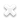Categories
Email:# 5A Lithium Charger CV CC buck Step down Power Supply

Power efficient switched mode power supply for use in your projects.
SKU: BAT-01502
Availability: Out of stock
R69,58
:

#### Description

Power efficient switched mode power supply for use in your projects.

#### Features

• Input voltage range:6 to 38VDC(Note:input voltage not exceeding 38V)
• Output current: 0-5A
• Output power: 75W
• High efficiency up to 96%
• Built in thermal shutdown function
• Built in current limit function
• Built in output short protection function
• Input reverse polarity protection: None (if required, high current diode in series with the input).
• Dimensions: L x W x H =61.7*26.2*15mm
• Weight: 20g

#### Application Ideas

A. Use as a step-down modules with over current protection capabilities

1. Adjust the "voltage potentiometer" so that the output voltage reaches the value you want.
2. Use a multimeter to measure the output short-circuit current with 10mA intervals, while regulating the "current potentiometer" so that the output current reaches a predetermined over current protection value. (For example, the meter displays the current value of 4A, then you can use the module to a maximum current of 4A)

B. Use as a battery charger

1. Make sure you need to charge the battery float voltage and charging current; (if lithium parameters 3.7V/2200mAh, then the float voltage is 4.2V, the maximum charging current 1C, ie 2200mA)
2. Under no-load conditions, adjust the "Voltage potentiometer" so that the output voltage reaches the float voltage; (if to 3.7V rechargeable lithium battery, the output voltage can be adjusted to 4.2V)
3. Use a multimeter to measure the output short-circuit current with 10mA intervals, while regulating the "current potentiometer" so that the output current reaches a predetermined Charging current value.
4. Charge turn lamp current factory default is 0.1 times the charging current; (Battery during charging current is gradually reduced, if the charge current setting is 1A, then when the charge current is less than 0.1A, blue lights turned off, the green light is on, which means that the battery is fully charged)
5. Connect to the battery.

(1,2,3,4 steps as: Output is unloaded, do not connect the battery)

C. Use as a LED constant current driver module

1. Adjust the "voltage potentiometer" so that the output voltage reaches the value you want.
2. Use a multimeter to measure the output short-circuit current with 10A intervals, while regulating the "current potentiometer" so that the output current reaches a predetermined LED operating current.
3. Connect LED, work.

(1,2 steps as: Output is unloaded, do not connect LED)

Customers who bought this item also bought